AP State Syllabus AP Board 6th Class Maths Solutions Chapter 3 HCF and LCM Unit Exercise Textbook Questions and Answers.

## AP State Syllabus 6th Class Maths Solutions 3rd Lesson HCF and LCM Unit Exercise

Question 1.
Classify the given numbers according to their divisibility.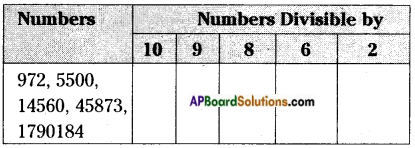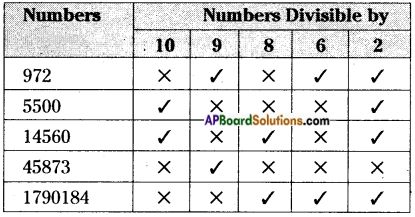(OR)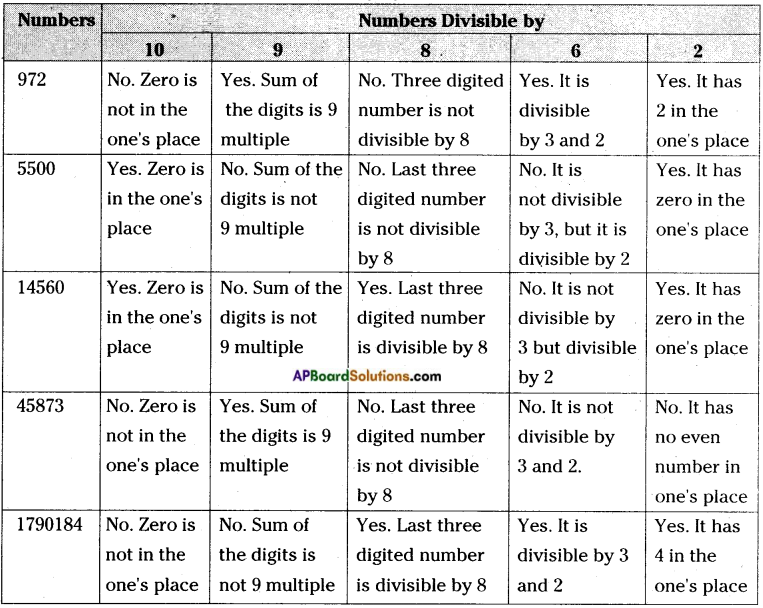Question 2.
Write the divisibility rule by 11 with one example.
Divisibility by 11:
A given number is divisible by 11, if the difference between the sum of the digits at odd places and the sum of the digits at even places (from the right) is either ‘O’ or a multiple of 11.
Ex: 123321
Sum of the digits at odd places = 1 + 3 + 2 = 6.
Sum of the digits at even places = 2 + 3 + 1 = 6
Their difference = 6 – 6 = 0
So, 123321 is divisible by 11.

Question 3.
Fill the table with correct answer.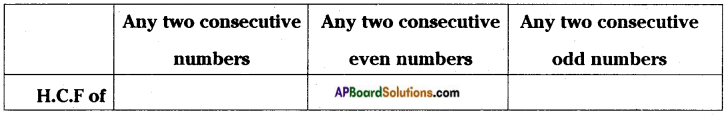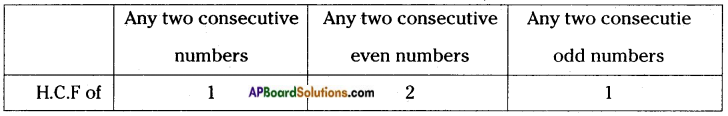Question 4.
Find HCF of 70, 105 and 175 by prime factorization method.
Given numbers are 70, 105 and 175.
The HCF of 70, 105 and 175 can be formed by prime factorization as follows:Thus, 70 = 2 × 5 × 7
105 = 3 × 5 × 7
175 = 5 × 5 × 7
Common factors of 70, 105 and 175 are: 5, 7
Their product: 5 × 7 = 35
Hence, HCF of 70, 105 and 175 is 35.Question 5.
Find HCF of 18, 54, 81 by continued division method.
Given numbers are 18, 54 and 81 To find the HCF of 18, 54 and 81
First find the HCF of 18 and 54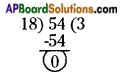HCF of 18 and 54 is 18.
Then find the HCF of the third number and the HCF of first two numbers.
i.e., let us find the HCF of 81 and 18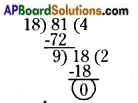Last divisor is 9 when remainder is zero.
∴ HCF of 18, 54 and 81 is 9.

Question 6.
Find LCM of 4, 12, 24 by two methods.
Given numbers are 4, 12 and 24
a) LCM by prime factors method:
Factors of 4 = 2 × 2
Factors of 12 = 2 × 2 × 3
Factors of 24 = 2 × 2 × 2 × 3
LCM of 4, 12 and 24= 2 × 2 × 2 × 3 = 24

b) Division method: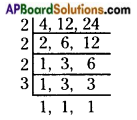Thus, the LCM of 4, 12 and 24 is 2 × 2 × 2 × 3 = 24Question 7.
What is the capacity of the largest vessel which can empty the oil from three vessels containing 32 liters, 24 liters and 48 liters respectively, an exact number of times?
Given capacity of three vessels are 32 liters, 24 liters and 48 liters.
To find the capacity of the largest vessel.
We have to find the HCF of 32, 24 and 48.
First find the HCF of 32 and 24.
HCF of 32 and 24 is 8.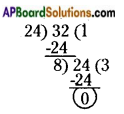Then, find the HCF of third number and the HCF of first two numbers.
i.e., let us find the HCF of 48 and 8.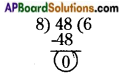HCF of 32, 24 and 48 is 8.
Hence, the capacity of the largest vessel which can empty the oil, then the three vessels containing 32 l, 24 l and 48 l of exact number of times is 8 liters.Question 8.
HCF, LCM of two numbers are 9 and 54 respectively. If one of those two numbers is 18, find the other number.
b = $$\frac{54 × 9}{18}$$ = 27